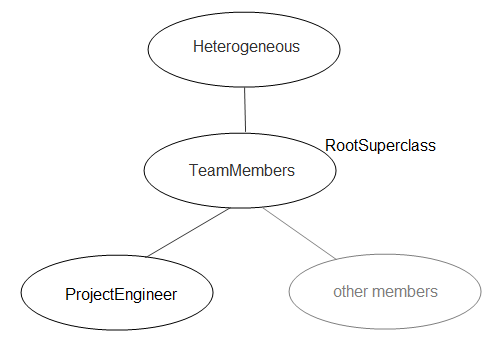## Heterogeneous Array Constructors

### Building Arrays in Superclass Constructors

When a subclass in a heterogeneous class hierarchy calls its superclass to construct an array of objects, you must ensure that the superclass constructor does not return a heterogeneous array to the subclass. The following programming patterns show how to avoid the errors caused by returning the wrong class to the subclass constructor.

### When Errors Can Occur

Constructors must return objects that are the same class as the defining class. When working with objects from a heterogeneous class hierarchy, the class of an object array can change as you add array elements of different classes. As a result, heterogeneous superclass constructors can change the class of object arrays when the class design requires all the following techniques:

• Building object arrays in subclass constructors

• Calling superclass constructors from subclass constructors to pass arguments

• Creating object arrays in the superclass constructor

In addition, either of the following is true:

• The root superclass is not abstract and does not implement a `getDefaultScalarElement` method.

• The root superclass implements a `getDefaultScalarElement` method that returns an object that is not the same class as the subclass.

When assigning to object arrays, MATLAB® uses the default object to fill in unassigned array elements. In a heterogeneous hierarchy, the default object can be the superclass that is called by the subclass constructor. Therefore, building an array in the superclass constructor can create a heterogeneous array.

If a superclass constructor returns a heterogeneous array to the subclass constructor, MATLAB generates an error (see Potential Error).

### Initialize Array in Superclass Constructor

To avoid errors, initialize the object array explicitly in the superclass constructor. For example, use `repelem` in the superclass constructor to initialize the array before initializing the superclass part of the objects. Initializing the array ensures that all elements assigned into the array are of the same class as the `obj` argument.

In this code, the superclass constructor creates one object for each element in the input argument, `arg`:

```method function obj = SuperClass(arg) ... n = numel(arg); obj = repelem(obj,1,n); for k = 1:n obj(k).SuperProp = arg(k); end ... end end```

The subclass constructor calls the superclass constructor to pass the required argument array, `a`:

```method function obj = SubClass(a) obj = obj@SuperClass(a); for k = 1:numel(a) obj(k).SubProp = a(k); end end end```

### Sample Implementation

The following class hierarchy defines a subclass that builds object arrays in its constructor. The root superclass of the hierarchy initializes the superclass part of the objects in the array.

This class hierarchy represents members of an engineering team. The classes in the hierarchy include:

• `TeamMembers` — Superclass for specific team member classes, like `ProjectEngineer`. `TeamMembers` defines the `Name` and `PhoneX` properties and derives from `matlab.mixin.Heterogeneous`.

• `ProjectEngineer` — Team members that are engineers. Each instance inherits a `Name` and `PhoneX` property and defines a billing `Rate` property.

• Other members — Other types of team members not implemented for this example for simplicity.The `TeamMembers` class is the root of the heterogeneous hierarchy and is a concrete class. Before assigning values to the `Name` and `PhoneX` properties, the constructor initializes an array of subclass (`ProjectEngineer`) objects.

The `ProjectEngineer` constructor provides the `obj` argument for the call to `repelem` with this statement:

`obj = obj@TeamMembers(varargin{1:2});`

Here is the `TeamMembers` class:

```classdef TeamMembers < matlab.mixin.Heterogeneous properties Name PhoneX end methods function obj = TeamMembers(nme,ext) if nargin > 0 n = numel(nme); obj = repelem(obj,1,n); for k = 1:n obj(k).Name = nme{k}; obj(k).PhoneX = ext(k); end else obj.Name = ''; end end end end```

The `ProjectEngineer` class represents one type of team member. This class supports array inputs and returns an array of objects.

```classdef ProjectEngineer < TeamMembers % Inputs: {Name}, [PhoneX], {Rate} properties Rate end methods function obj = ProjectEngineer(varargin) obj = obj@TeamMembers(varargin{1:2}); for k = 1:numel(varargin{1}) obj(k).Rate = varargin{3}{k}; end end end end```

The `ProjectEngineer` class requires a cell array of names, a numeric array of phone extensions, and a cell array of billing rates for each engineer in the team.

```nm = {'Fred','Nancy','Claudette'}; px = [8112,8113,8114]; rt = {'C2','B1','A2'}; tm = ProjectEngineer(nm,px,rt)```
```tm = 1x3 ProjectEngineer array with properties: Rate Name PhoneX```

### Potential Error

The `TeamMembers` constructor initializes the object array with this statement:

`obj = repelem(obj,1,n);`

Because the `obj` argument to `repelem` is a `ProjectEngineer` object, the array returned is of the same class.

Without this statement, the `TeamMembers` constructor would create default objects to fill in array elements in the `for` loop. The resulting heterogeneous array would be of the class of the common superclass (`TeamMembers` in this case). If the superclass returns this heterogeneous array to the subclass constructor, it is a violation of the rule that class constructors must preserve the class of the returned object.

MATLAB issues this error:

```When constructing an instance of class 'ProjectEngineer', the constructor must preserve the class of the returned object. Error in ProjectEngineer (line 8) obj = obj@TeamMembers(varargin{1:2}); ```